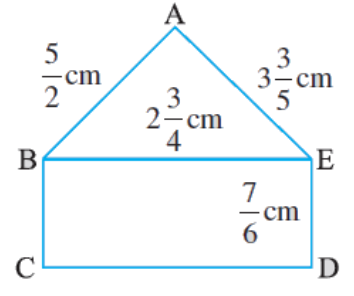# Ex 2.1 Q5 Fractions and Decimals Solution - NCERT Maths Class 7

## Question

Find the perimeter of

i)$$∆ABE$$

ii) The rectangle $$BCDE$$ in this figure. Whose perimeter is greater?Video Solution
Fractions And Decimals
Ex 2.1 | Question 5

## Text Solution

What is Known?

A rectangle and a triangle

What is unknown?

Perimeter of the given rectangle and triangle.

Reasoning:

Perimeters of the rectangle and triangle can be calculated by adding lengths of four sides of the rectangle and the three sides of the triangle. Perimeters can be compared to decide whose perimeter is greater.

Steps:

i) Perimeter of $$∆ABE$$ $$=$$ sum of all sides $$=$$ $$AB + BE + AE$$

\begin{align} & =\frac{5}{2}\text{cm}+2\frac{3}{4}\text{cm}+3\frac{3}{5}\text{cm} \\ & =\left( \frac{5}{2}+2\frac{3}{4}+3\frac{3}{5} \right)\text{cm} \\ & =\left( \frac{5}{2}+\frac{11}{4}+\frac{18}{5} \right)\text{cm} \\ & =\left( \frac{50+55+72}{20} \right)\text{cm}\\&=\frac{177}{20}\text{cm}\\& =8\frac{17}{20}\text{cm}\end{align}

Therefore, perimeter of $$∆ABE$$  is \begin{align}8\frac{{17}}{{20}}{\rm{cm}}\end{align}

ii) In rectangle, $$BCDE$$

\begin{align}{\rm{BE}} = 2\frac{3}{4}{\rm{cm}},{\rm{ED}} = \frac{7}{6}{\rm{cm}}\end {align}

Perimeter of rectangle $$BCDE = 2 \text{(Length + Breadth)}$$

\begin{align} & ={ 2\left( {2\frac{3}{4} + \frac{7}{6}} \right)}\\ & ={ 2\left( {\frac{{11}}{4} + \frac{7}{6}} \right)}\\ &= {2\left( {\frac{{66 + 28}}{{24}}} \right)}\\&= {2\left( {\frac{{94}}{{24}}} \right)}\\&= {\frac{{47}}{6}}\\&={ 7\frac{5}{6}{\rm{cm}}}\end{align}

Therefore, perimeter of rectangle $$BCDE$$  is \begin{align}7\frac{5}{6}{\rm{cm}}.\end{align}

On comparing,

\begin{align}8\frac{{17}}{{20}}{\rm{cm}}\end{align}> \begin{align}7\frac{5}{6}{\rm{cm}}\end{align}

Therefore, perimeter of $$∆ABE$$ is greater than the perimeter of rectangle $$BCDE$$.

Learn from the best math teachers and top your exams

• Live one on one classroom and doubt clearing
• Practice worksheets in and after class for conceptual clarity
• Personalized curriculum to keep up with school